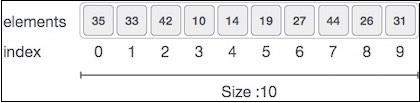# Arrays Data Structure in Javascript

The array is a container which can hold a fixed number of items and these items should be of the same type. It stores a fixed-size sequential collection of elements of the same type. An array is used to store a collection of data, but it is often more useful to think of an array as a collection of variables of the same type.

## Why do we need arrays?

Let's say you want to record the average temperatures of all days of the week. You can record them as follows −

let avgTempMon = 35;
let avgTempTue = 33;
let avgTempWed = 31;
let avgTempThur = 24;
let avgTempFri = 25;
let avgTempSat = 22;
let avgTempSun = 30;

But if you look at this it gets difficult to keep track of these variables. What if you had to do this for all months? It would get very difficult to keep track. So we use arrays to keep track of these −

let avgTemps = [];
avgTemps = 35;
avgTemps = 33;
avgTemps = 31;
avgTemps = 24;
avgTemps = 25;
avgTemps = 22;
avgTemps = 30;

Now you only need to keep track of one variable to get all the values.

## Arrays Representation

Arrays are represented as contiguous blocks of memory holding data in them. For example,The above image shows an array of 10 elements. The indices are the places where each of these elements are stored. Note that index starts with 0 and each element can be accessed via its index. For example, we can fetch an element at index 6 as arr which will give the result 27.

## Operations on Arrays

Let's look at some of the operations that can be applied to arrays in Javascript.

• Creating an array